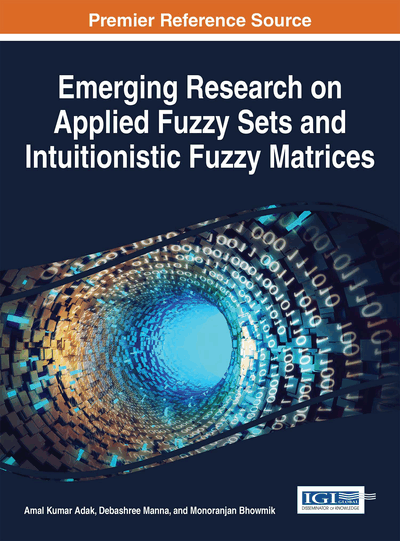# An Efficient Method for Forecasting Using Fuzzy Time Series

Pritpal Singh (CHARUSAT University, India)
DOI: 10.4018/978-1-5225-0914-1.ch013
Available
\$37.50
No Current Special Offers

## Abstract

Forecasting using fuzzy time series has been applied in several areas including forecasting university enrollments, sales, road accidents, financial forecasting, weather forecasting, etc. Recently, many researchers have paid attention to apply fuzzy time series in time series forecasting problems. In this paper, we present a new model to forecast the enrollments in the University of Alabama and the daily average temperature in Taipei, based on one-factor fuzzy time series. In this model, a new frequency based clustering technique is employed for partitioning the time series data sets into different intervals. For defuzzification function, two new principles are also incorporated in this model. In case of enrollments as well daily temperature forecasting, proposed model exhibits very small error rate.
Chapter Preview
Top

## 1. Introduction

Forecasting using fuzzy time series has been applied in several areas including forecasting university enrollments, sales, road accidents, financial forecasting, weather forecasting, etc. In a conventional time series, the recorded values of a special dynamic process are represented by crisp numerical values. However, in a fuzzy time series concept, the recorded values of a special dynamic process are represented by linguistic values. Based on fuzzy time series concept, first forecasting model was introduced by Song and Chissom (Song & Chissom, 1993,1994,1993). They presented the fuzzy time series model by means of fuzzy relational equations involving max-min composition operation and applied the model to forecast the enrollments in the University of Alabama. Chen (1996) used simplified arithmetic operations avoiding the complicated max-min operations and their method produced better results. Later, many studies provided some improvements to the existing methods in terms of effective lengths of intervals, fuzzification and defuzzification techniques used.

Hwang et al. (1998) used the differences of the available historical data as fuzzy time series instead of direct usage of raw numeric values. Sah and Degtiarev also used a similar approach in this article (Sah & Konstantin, 2005). Huarng tried to improve the forecasting accuracy based on the determination of effective length of intervals (Huarng, 2001a), and heuristic approaches (Huarng, 2001b). In this article (Lee& Chou, 2004), authors forecasted the university enrollments with the average error rate less than Chen's method (Chen, 1996) by defining the supports of the fuzzy numbers that represent the linguistic values of the linguistic variables more appropriately.

Cheng et al. (2006)used entropy minimization to create the intervals. They also used trapezoidal membership functions in the fuzzification process. Chang et al. (2007) presented cardinality-based fuzzy time series forecasting model, which builds weighted fuzzy rules by calculating the cardinality of fuzzy relations. To enhance the performance of fuzzy time series models, Chen et al. (2007) incorporates the concept of the Fibonacci sequence in the existing models as proposed by Song and Chissom (Song & Chissom, 1993,1994,1993), and Yu (Yu, 2005). To obtain less number of intervals, Cheng et al. (2008) proposed a model using fuzzy clustering technique to partition the data effectively. The k-means clustering algorithm has been applied to partition the universe of discourse in fuzzy time series modeling approach (Kai et al. 2010). Chou et al. (2010) forecasted the tourism demand based on hybridization of rough set with fuzzy time series. In this article (Singh & Borah, 2011), authors forecasted the university enrollments with the help of new proposed algorithm by dividing the universe of discourse of the historical time series data into different length of intervals.

## Complete Chapter List

Search this Book:
Reset